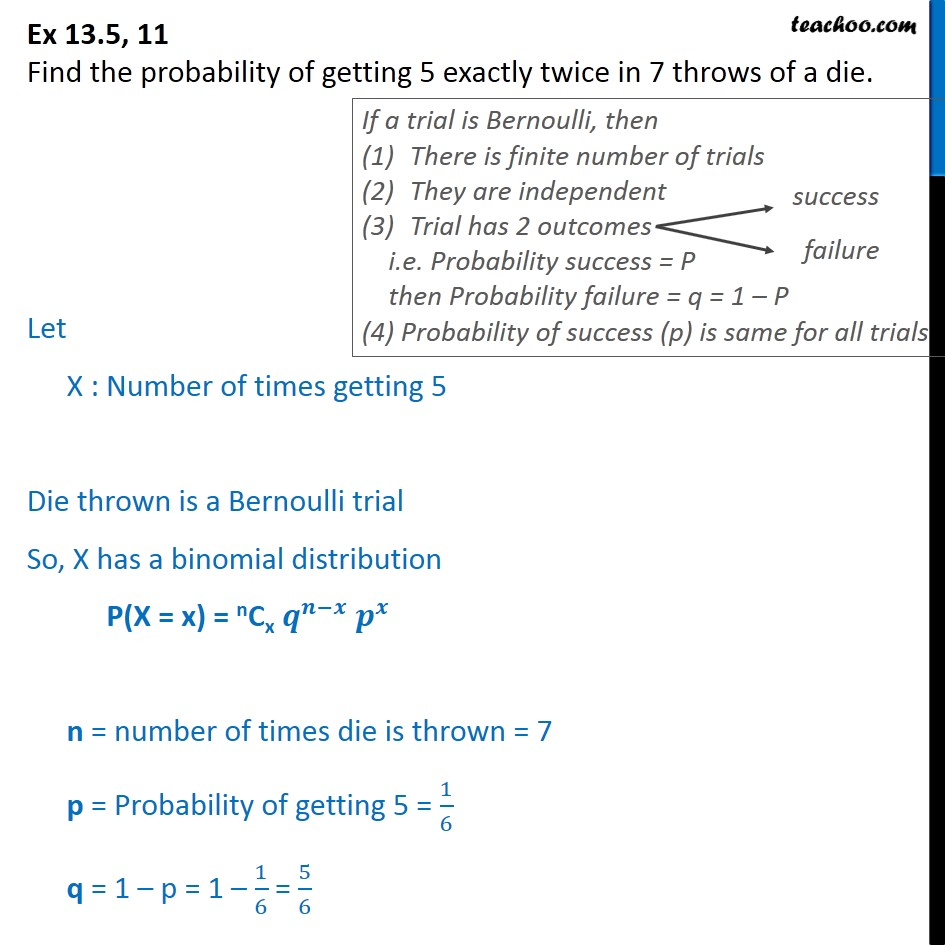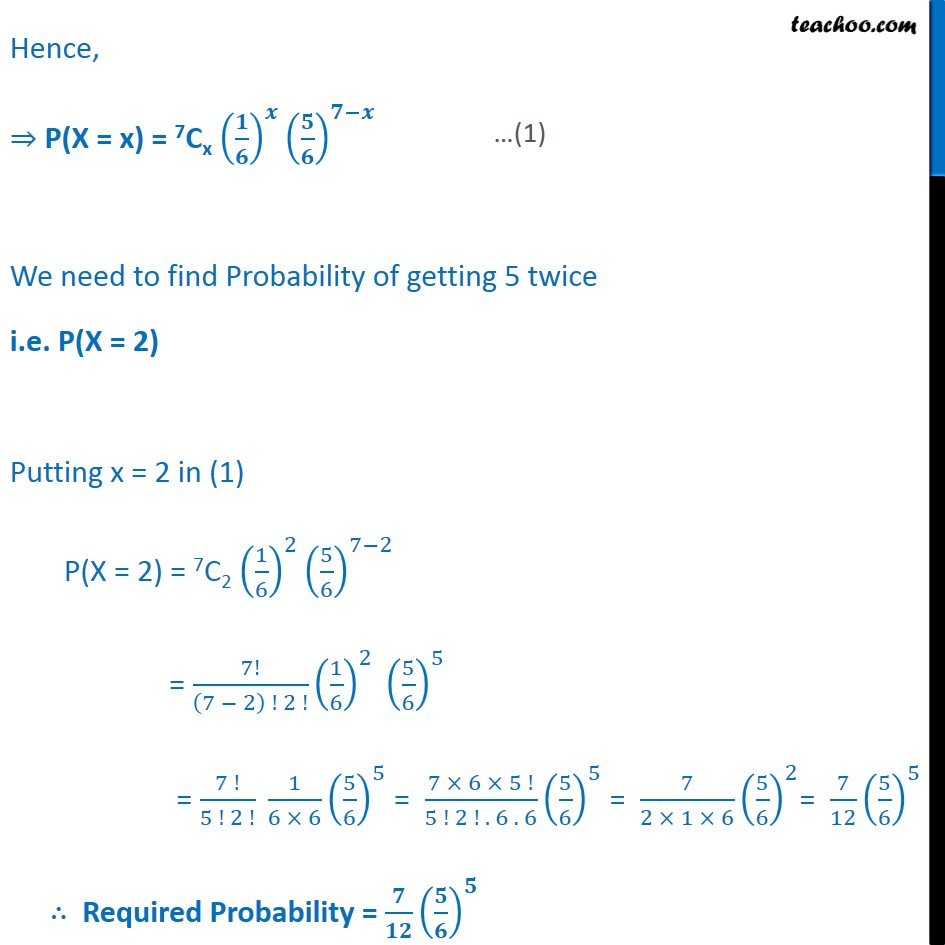Bernoulli Trial

Chapter 13 Class 12 Probability
Serial order wiseLearn in your speed, with individual attention - Teachoo Maths 1-on-1 Class

### Transcript

Question 11 Find the probability of getting 5 exactly twice in 7 throws of a die. Let X : Number of times getting 5 Die thrown is a Bernoulli trial So, X has a binomial distribution P(X = x) = nCx n = number of times die is thrown = 7 p = Probability of getting 5 = 1 6 q = 1 p = 1 1 6 = 5 6 Hence, P(X = x) = 7Cx We need to find Probability of getting 5 twice i.e. P(X = 2) Putting x = 2 in (1) P(X = 2) = 7C2 1 6 2 5 6 7 2 = 7! 7 2 ! 2 ! 1 6 2 5 6 5 = 7 ! 5 ! 2 ! 1 6 6 5 6 5 = 7 6 5 ! 5 ! 2 ! . 6 . 6 5 6 5 = 7 2 1 6 5 6 2 = 7 12 5 6 5 Required Probability =